### Math History

In the $19^\text{th}$ century B.C., the pharaoh Amenemhat III ordered the construction of several pyramids. Like other pharaohs, Amenemhat hoped the monuments constructed during his reign would grant him honor and immortality.

As with all grand construction projects, the construction of these pyramids required a significant amount of planning and computation, much of it complicated and new. In reality, it's the work of Amenemhat's scribes and engineers that has lasted — far longer than his pyramids, which are mostly ruins now.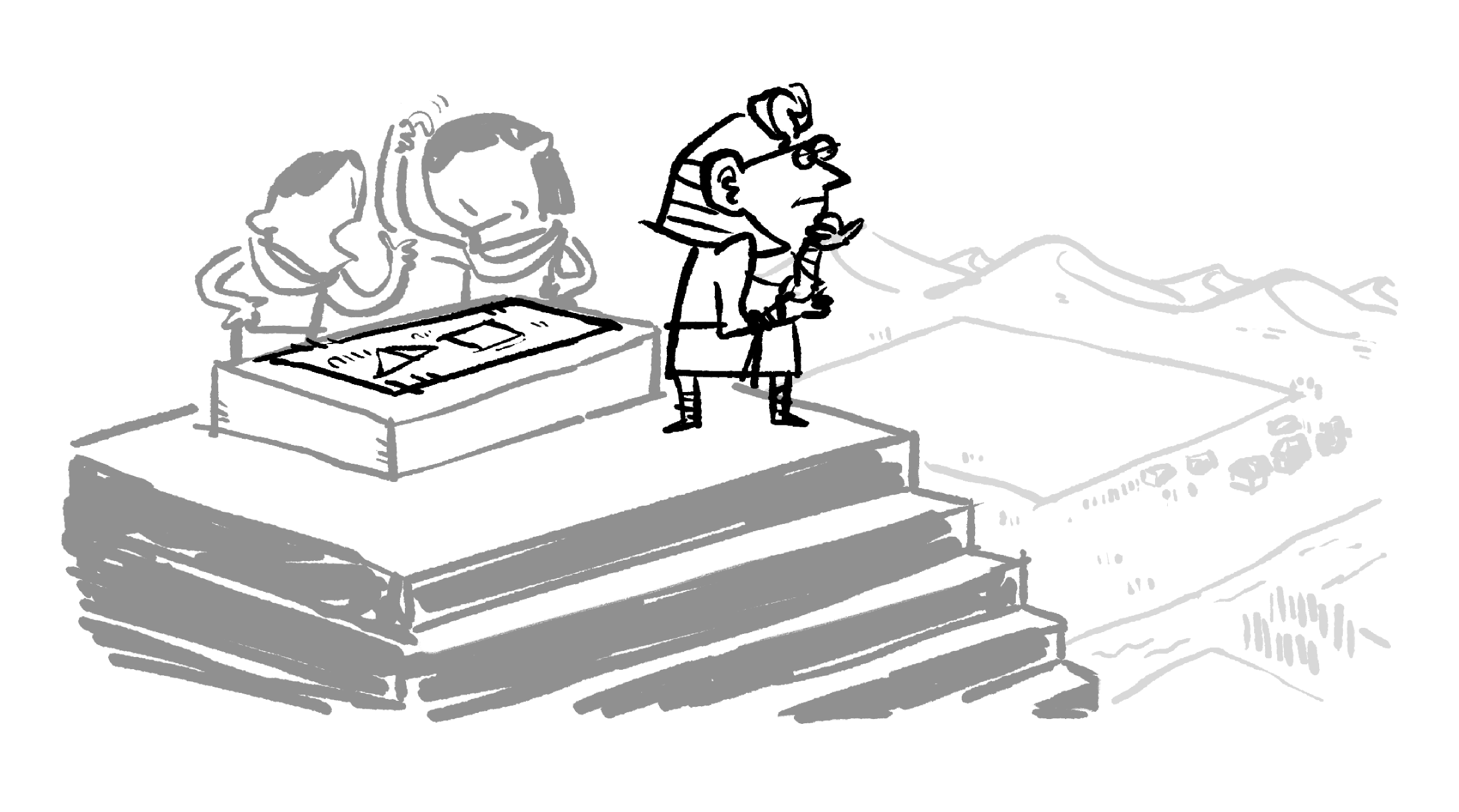While we don't know the names of those original mathematical idea-builders, a mathematician who copied their work a few centuries later was named Ahmes. It's from his writing that we know most of what we do about ancient Egyptian mathematics.

# Egyptian Multiplication

Like the other scribes of his day, Ahmes probably spent a lot of his time doing calculations because there were no electronic computers or calculators. All calculations had to be done by hand, and much of that depended on clever algorithms.

The Egyptians did not have a technique for multiplying numbers directly as we do. Instead, they used doubling, which can be performed by adding a number to itself.

If you're clever about it — and Ahmes and his compatriots were! — you can use addition and doubling to multiply any two numbers together.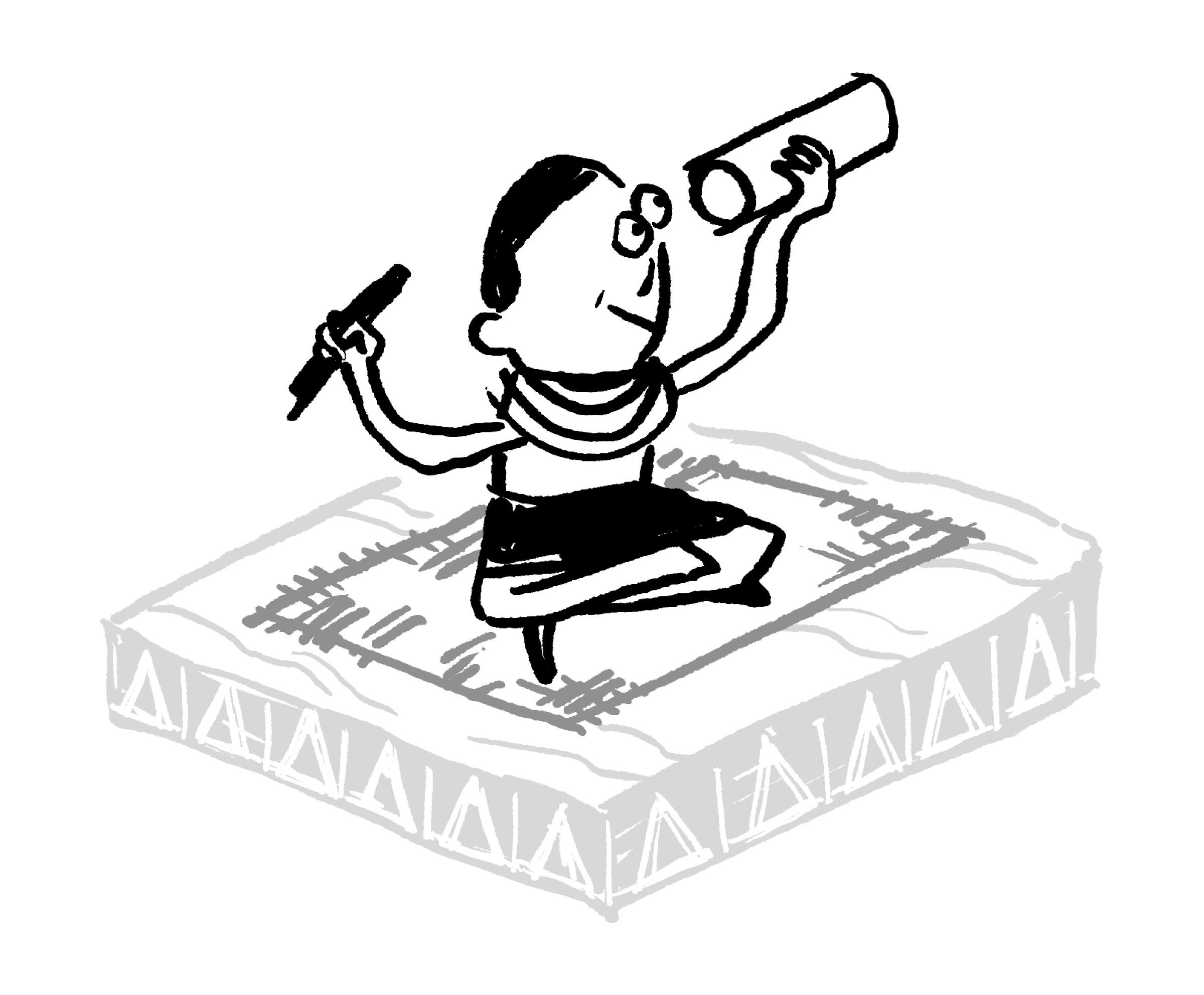# Egyptian Multiplication

Let's lay some of the foundations for how Ahmes's method of multiplying worked.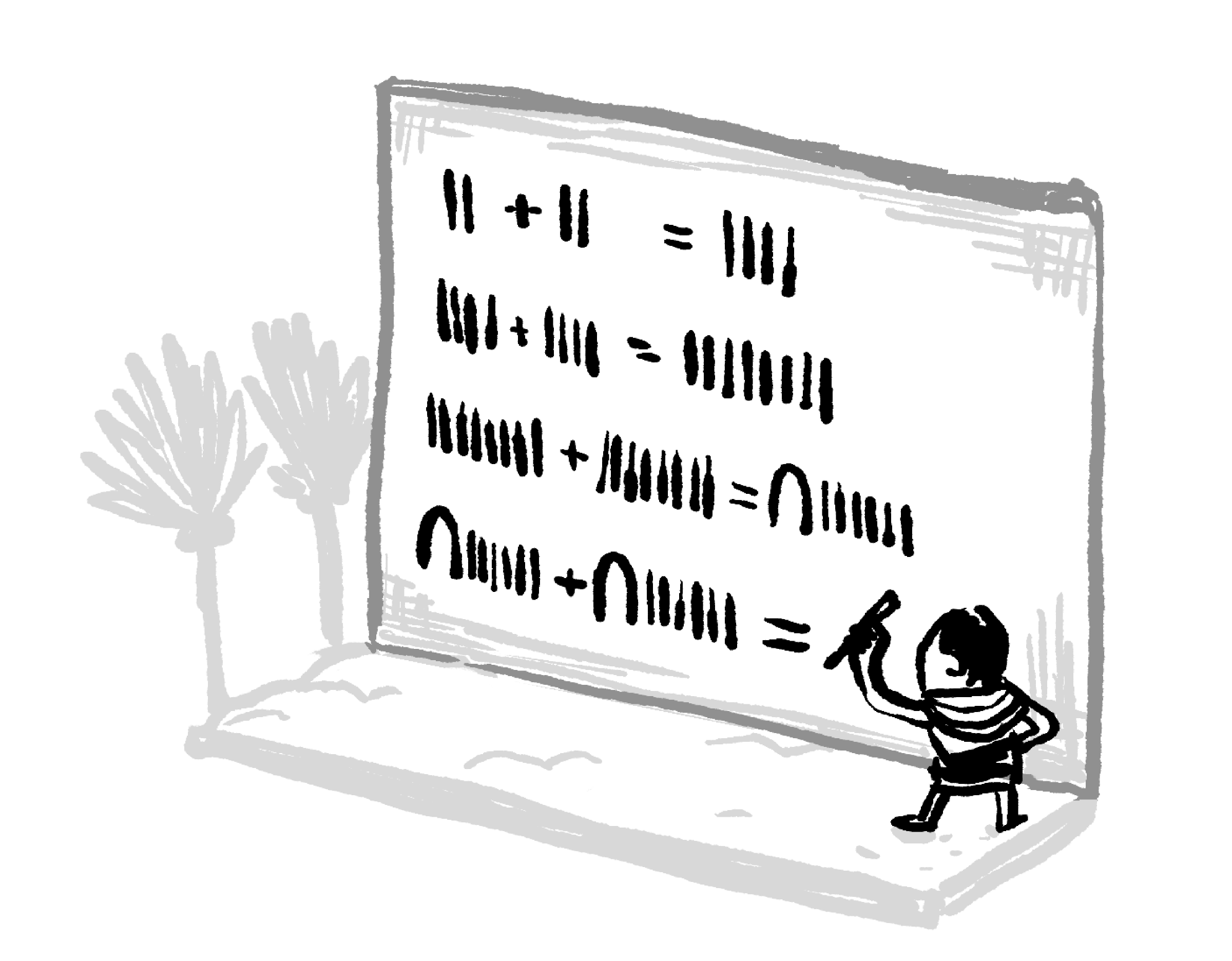The top row here is $2+2=4$ in Egyptian numerals; it's not saying that $11+11=1111.$

Starting with $1$ and doubling repeatedly, we get $1, 2, 4, 8, 16, \dots$

Select numbers from this list that together add up to $13,$ using the least amount of numbers possible.

# Egyptian Multiplication

Select one or more

To use Ahmes's method for multiplying two numbers, we begin by constructing a table of values.

To multiply $13 \times 24$, we'll need two columns: one on the left, starting with one, and one on the right, starting with $24$. We double the numbers in both columns repeatedly until we've exceeded $13$ on the left.

Then, since we want to multiply $24$ by $13$, we choose values in the left column that add to $13:$

Adding together the multiples of $24$ that matched up with the numbers that added to $13$ gives us the product of $13$ and $24.$

The result is $24 + 96 + 192 = 312,$ which is the correct value of $13 \times 24$. So, this method works, but why?

# Egyptian Multiplication

Let's use Ahmes's method to calculate $17 \times 31.$

Egyptian Multiplication

1. Construct a table of doubles starting with $1$ on the left and the number to be multiplied on the right.
2. Select values on the left that add to the number of times we want to multiply.
3. Add the right column values that match up with Step $2.$
 $1$ $31$ $2$ $62$ $4$ $124$ $8$ $248$ $16$ $496$ $32$ $992$

What is $17 \times 31?$

# Egyptian Multiplication

Try using Ahmes's method to calculate $24 \times 21$. This time, you'll need to make the table yourself.

Egyptian Multiplication

1. Construct a table of doubles $($with $1$ on the left and the number to be multiplied on the right$).$
2. Select values on the left that add to the number of times we want to multiply.
3. Add the right column values that match up with Step $2.$

What is $24 \times 21?$

# Egyptian Multiplication

We're now going to shift our focus to the question of why. That is, why does this procedure produce correct results for multiplication?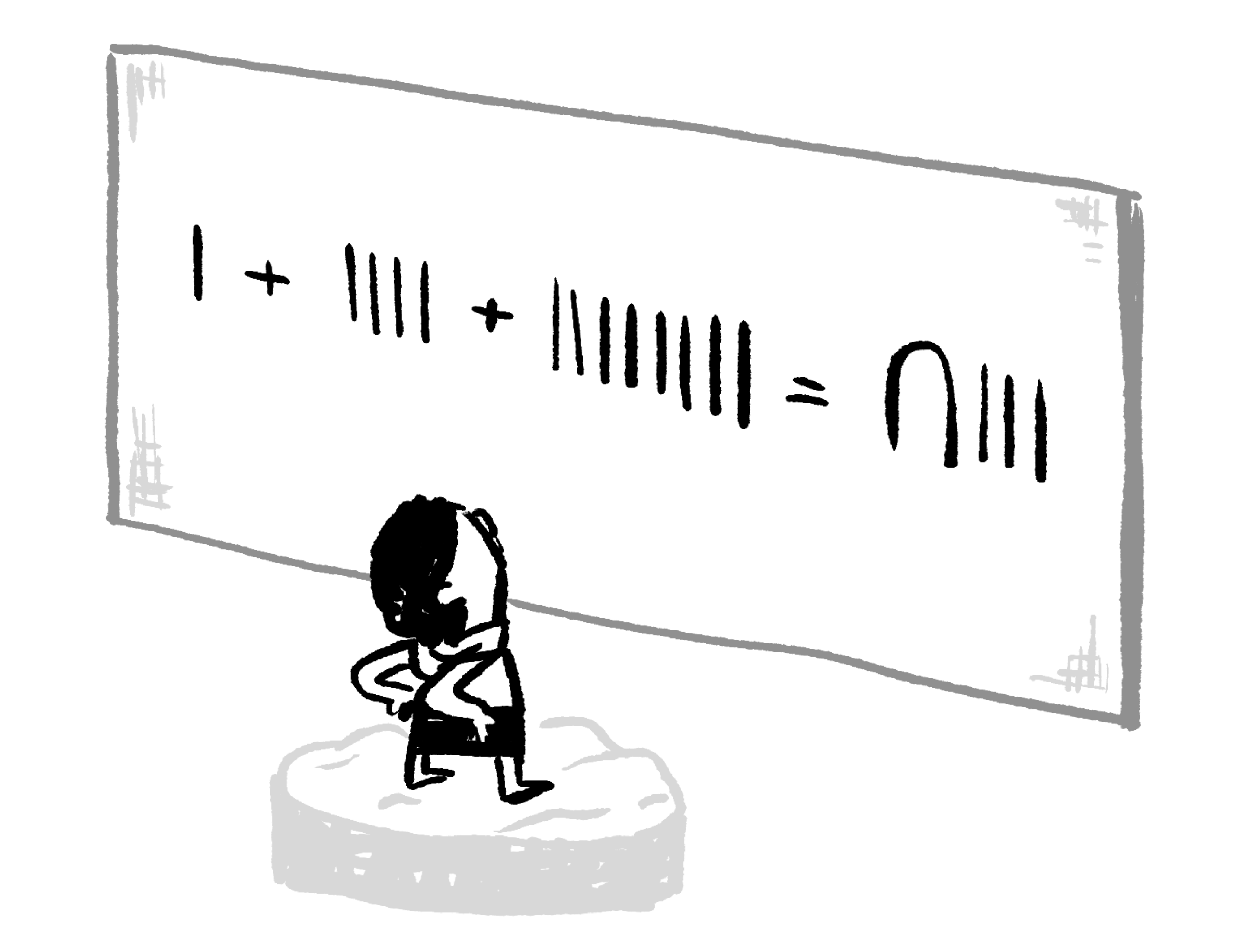The first step of Ahmes's algorithm depends on writing numbers as a sum of powers of two. For example, we rewrote $13$ as $1 + 4 + 8.$

How hard is it to break down a number like that, though? In the interactive below, you can drag the powers of two and drop them in the boxes. See if you can build each sum.

# Egyptian Multiplication

In fact, every positive integer can be written as a sum of distinct powers of two. Because of this, Ahmes's approach can be used to multiply any two positive integers together.

This ancient algorithm for multiplying two numbers is somewhat similar to the way modern computers multiply, because Ahmes's algorithm basically converts one of the numbers into base two (or binary).

Later in this course, we'll meet Ada Lovelace, one of the first people to write computational instructions for machines. She probably would have enjoyed meeting Ahmes, but their lives were separated by dozens of centuries.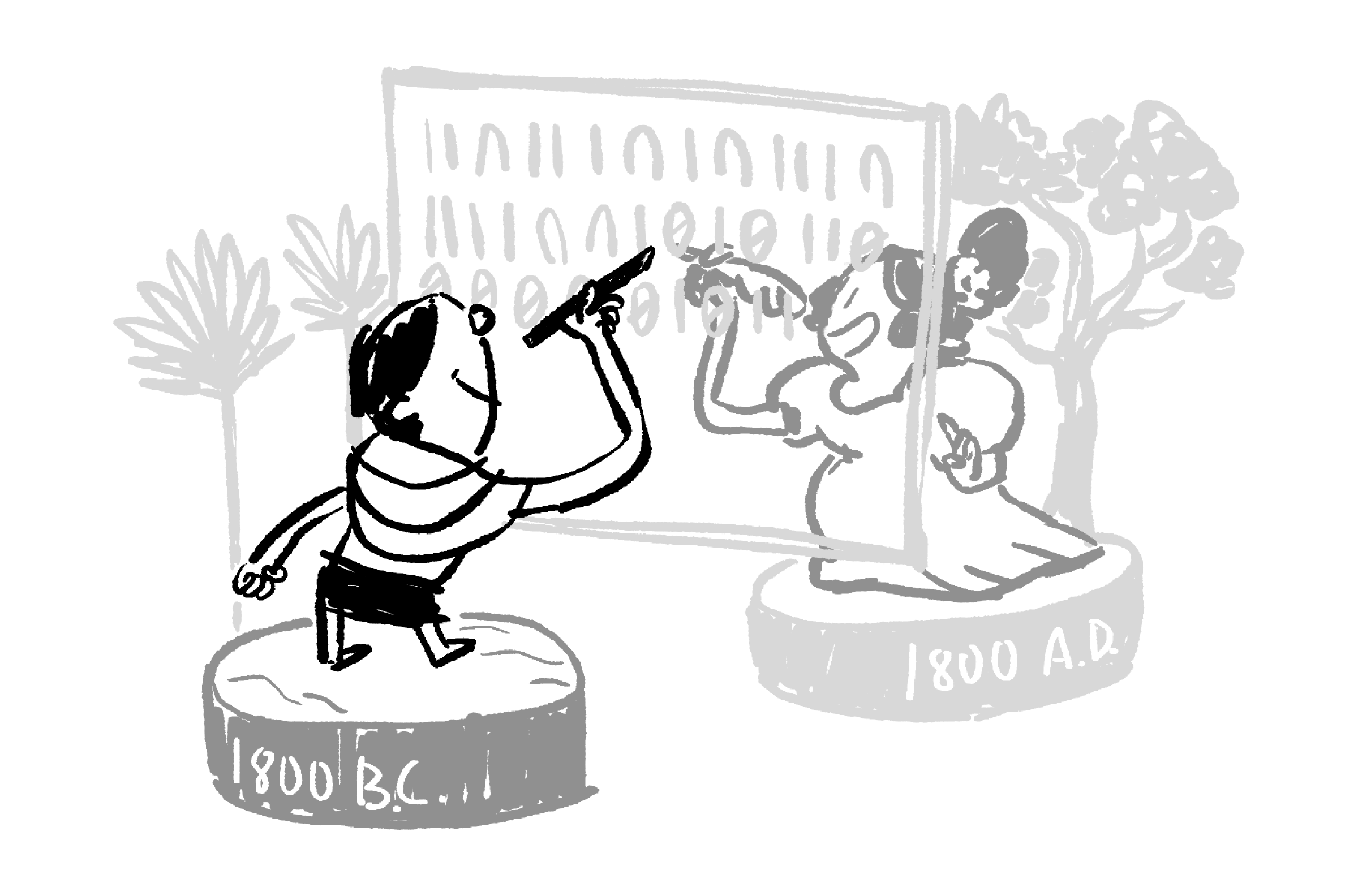# Egyptian Multiplication

Despite its relationship to binary, however, we don't need to use base two to understand how Ahmes's approach to multiplication works. Let's look at our first problem again, $13 \times 24.$

We selected the following numbers to add, because $1 + 4 + 8 =13:$

 $\color{#D61F06} 1$ $\color{#D61F06} 24$ $2$ $48$ $\color{#D61F06} 4$ $\color{#D61F06} 96$ $\color{#D61F06} 8$ $\color{#D61F06} 192$ $16$ $384$

Which expression is equivalent to $24 + 96 + 192?$

A) $\ 1 \times 24 + 4 \times 24 + 8 \times 24$

B) $\ 2 \times (12+ 24 + 48)$

C) $\ 4 \times 13 + 4 \times 24 + 4 \times 96$

# Egyptian Multiplication

Ahmes's doubling technique turned the problem $13 \times 24$ into the addition problem $24 + 96 + 192$ by making use of this table:

We can see why this process works by factoring the values in the addition problem: \begin{aligned} 24 + 96 + 192 &= 1 \times 24 + 4 \times 24 + 8 \times 24 \\ &= (1 + 4 + 8) \times 24 \\ &= 13 \times 24. \end{aligned}

# Egyptian Multiplication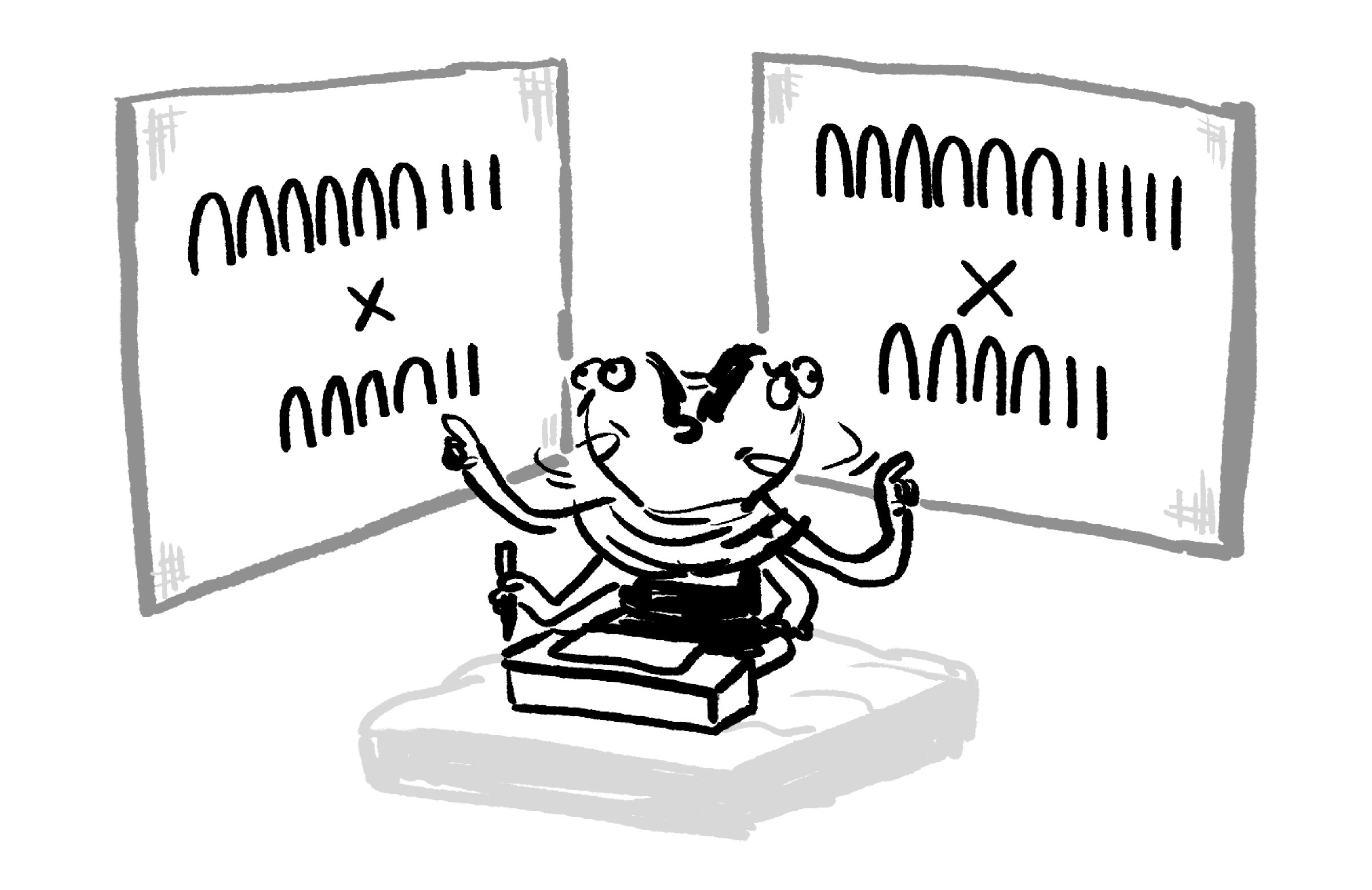If you use Ahmes's method of multiplication on both products, which requires the least addition in the final step, $63 \times 42 \quad \text{or}\quad 65 \times 42?$

# Egyptian Multiplication

Ahmes is the very first mathematician whose name we know, and what we actually remember him for is mostly duplication of work from hundreds of years before his time.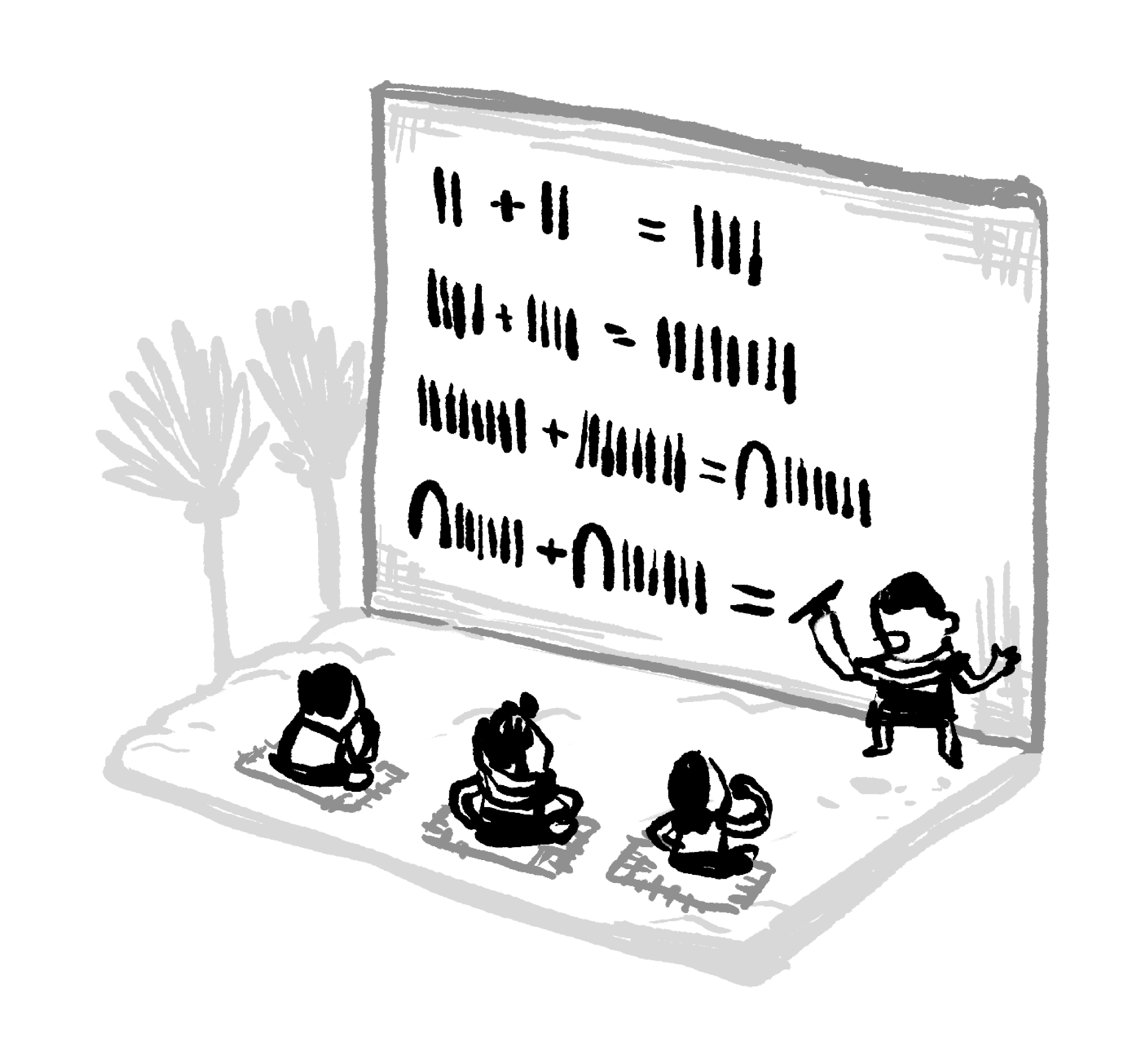Although this approach to multiplication might seem fairly simple to us, it has connections that reach both forward and backward in time. Egyptian multiplication is connected to many ideas that still intrigue and puzzle us today, but its primary importance in Ahmes's time was as a tool.

By turning multiplication into addition, this powerful technique made otherwise intractable problems into something that anyone with basic training could accomplish.

# Egyptian Multiplication

×